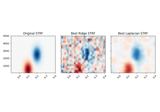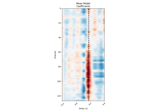# mne.decoding.ReceptiveField¶

class mne.decoding.ReceptiveField(tmin, tmax, sfreq, feature_names=None, estimator=None, fit_intercept=None, scoring='r2', patterns=False, n_jobs=1, edge_correction=True, verbose=None)[source]

Fit a receptive field model.

This allows you to fit an encoding model (stimulus to brain) or a decoding model (brain to stimulus) using time-lagged input features (for example, a spectro- or spatio-temporal receptive field, or STRF) 1234.

Parameters
tmin`float`

The starting lag, in seconds (or samples if `sfreq` == 1).

tmax`float`

The ending lag, in seconds (or samples if `sfreq` == 1). Must be >= tmin.

sfreq`float`

The sampling frequency used to convert times into samples.

feature_names`array`, shape (n_features,) | `None`

Names for input features to the model. If None, feature names will be auto-generated from the shape of input data after running `fit`.

estimator

The model used in fitting inputs and outputs. This can be any scikit-learn-style model that contains a fit and predict method. If a float is passed, it will be interpreted as the `alpha` parameter to be passed to a Ridge regression model. If `None`, then a Ridge regression model with an alpha of 0 will be used.

fit_intercept

If True (default), the sample mean is removed before fitting. If `estimator` is a `sklearn.base.BaseEstimator`, this must be None or match `estimator.fit_intercept`.

scoring[‘r2’, ‘corrcoef’]

Defines how predictions will be scored. Currently must be one of ‘r2’ (coefficient of determination) or ‘corrcoef’ (the correlation coefficient).

patternsbool

If True, inverse coefficients will be computed upon fitting using the covariance matrix of the inputs, and the cross-covariance of the inputs/outputs, according to 5. Defaults to False.

n_jobs

Number of jobs to run in parallel. Can be ‘cuda’ if CuPy is installed properly and `estimator is None`.

New in version 0.18.

edge_correctionbool

If True (default), correct the autocorrelation coefficients for non-zero delays for the fact that fewer samples are available. Disabling this speeds up performance at the cost of accuracy depending on the relationship between epoch length and model duration. Only used if `estimator` is float or None.

New in version 0.18.

verbose

If not None, override default verbose level (see `mne.verbose()` and Logging documentation for more).

Notes

For a causal system, the encoding model will have significant non-zero values only at positive lags. In other words, lags point backward in time relative to the input, so positive lags correspond to previous input time samples, while negative lags correspond to future input time samples.

References

1

Frédéric E. Theunissen, Stephen V. David, Nandini C. Singh, Ann Hsu, William E. Vinje, and Jack L. Gallant. Estimating spatio-temporal receptive fields of auditory and visual neurons from their responses to natural stimuli. Network: Computation in Neural Systems, 12(3):289–316, 2001. doi:10.1080/net.12.3.289.316.

2

Ben Willmore and Darragh Smyth. Methods for first-order kernel estimation: simple-cell receptive fields from responses to natural scenes. Network: Computation in Neural Systems, 14(3):553–577, 2003. doi:10.1088/0954-898X_14_3_309.

3

Michael J. Crosse, Giovanni M. Di Liberto, Adam Bednar, and Edmund C. Lalor. The multivariate temporal response function (mTRF) toolbox: a MATLAB toolbox for relating neural signals to continuous stimuli. Frontiers in Human Neuroscience, 2016. doi:10.3389/fnhum.2016.00604.

4

Christopher R. Holdgraf, Wendy de Heer, Brian Pasley, Jochem Rieger, Nathan Crone, Jack J. Lin, Robert T. Knight, and Frédéric E. Theunissen. Rapid tuning shifts in human auditory cortex enhance speech intelligibility. Nature Communications, 2016. doi:10.1038/ncomms13654.

5

Stefan Haufe, Frank Meinecke, Kai Görgen, Sven Dähne, John-Dylan Haynes, Benjamin Blankertz, and Felix Bießmann. On the interpretation of weight vectors of linear models in multivariate neuroimaging. NeuroImage, 87:96–110, 2014. doi:10.1016/j.neuroimage.2013.10.067.

Attributes
coef_array, shape ([n_outputs, ]n_features, n_delays)

The coefficients from the model fit, reshaped for easy visualization. During `mne.decoding.ReceptiveField.fit()`, if `y` has one dimension (time), the `n_outputs` dimension here is omitted.

patterns_array, shape ([n_outputs, ]n_features, n_delays)

If fit, the inverted coefficients from the model.

delays_`array`, shape (n_delays,), dtype `int`

The delays used to fit the model, in indices. To return the delays in seconds, use `self.delays_ / self.sfreq`

valid_samples_`slice`

The rows to keep during model fitting after removing rows with missing values due to time delaying. This can be used to get an output equivalent to using `numpy.convolve()` or `numpy.correlate()` with `mode='valid'`.

Methods

 `__hash__`(/) Return hash(self). `fit`(X, y) Fit a receptive field model. `get_params`([deep]) Get parameters for this estimator. Generate predictions with a receptive field. `score`(X, y) Score predictions generated with a receptive field. `set_params`(**params) Set the parameters of this estimator.
fit(X, y)[source]

Fit a receptive field model.

Parameters
X`array`, shape (n_times[, n_epochs], n_features)

The input features for the model.

y`array`, shape (n_times[, n_epochs][, n_outputs])

The output features for the model.

Returns
selfinstance

The instance so you can chain operations.

Examples using `fit`:get_params(deep=True)[source]

Get parameters for this estimator.

Parameters
deepbool, optional

If True, will return the parameters for this estimator and contained subobjects that are estimators.

Returns
params`dict`

Parameter names mapped to their values.

predict(X)[source]

Generate predictions with a receptive field.

Parameters
X`array`, shape (n_times[, n_epochs], n_channels)

The input features for the model.

Returns
y_pred`array`, shape (n_times[, n_epochs][, n_outputs])

The output predictions. “Note that valid samples (those unaffected by edge artifacts during the time delaying step) can be obtained using `y_pred[rf.valid_samples_]`.

Examples using `predict`:score(X, y)[source]

Score predictions generated with a receptive field.

This calls `self.predict`, then masks the output of this and `y` with ``self.valid_samples_`. Finally, it passes this to a `sklearn.metrics` scorer.

Parameters
X`array`, shape (n_times[, n_epochs], n_channels)

The input features for the model.

y`array`, shape (n_times[, n_epochs][, n_outputs])

Used for scikit-learn compatibility.

Returns
scores`list` of `float`, shape (n_outputs,)

The scores estimated by the model for each output (e.g. mean R2 of `predict(X)`).

Examples using `score`:set_params(**params)[source]

Set the parameters of this estimator.

The method works on simple estimators as well as on nested objects (such as pipelines). The latter have parameters of the form `<component>__<parameter>` so that it’s possible to update each component of a nested object.

Parameters
**params`dict`

Parameters.

Returns
instinstance

The object.

## Examples using `mne.decoding.ReceptiveField`¶